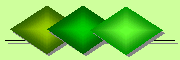De Claron LtdExpress Accountancy services at affordable prices
 Accounting Property Investment Reporting Company Secretarial ServicesHelp

Cash-on-Cash Return

Cash-on-Cash Return (COCR) is also referred to as equity dividend rate. It is a measure of a return received from property investments. Because financing of real estate acquisitions involves typically the use of both equity (one's own money) and debt (borrowed funds) the COCR takes into account loan repayments to derive net equity cash flow returns. That is, the income the investor receives net of debt service (loan or mortgage payments).

The formula for calculating the COCR is:

• COCR = BTECF / Equity investment

• where:

• BTECF = Net Operating Income (NOI) - Debt Service (loan or mortgage payments)
• Equity Investment = Total Investment Cost - Loan Amount
• To illustrate COCR formula we will consider a real estate investement with:

• total acquisition cost of \$1,000,000
• annual NOI of \$100,000
• \$600,000 mortgage loan with a 5% interest rate for a term of 20 years
• This gives an annual debt service of \$48,145.44 (if it is repaid annually).

The above assumes total monthly payment for a straight repayment mortgage, including both interest and payment towards the capital loan amount. This reduces the amount owed on the capital, month by month.

With these figures the COCR can be calculated as follows:

• BTECF = 100,000 - 48,145.44 = 51,854.56
• Equity Investment = 1,000,000 - 600,000 = 400,000
• With these two figures we can now estimate the cash-on-cash return as:

• COCR = 51,854.56 / 400,000 = 12.96%
• The higher the percentage figure then the higher cash flow is generated by the property. Hence, the property is potentially a good investment. However, COCR has the following limitations:

• The most important is that it does not account for potential changes in net operating income and property value over the years which maybe subject to appreciation or depreciation.
• Because COCR is based on before-tax cash flow over the amount of cash invested, it cannot take into account an individual investor's tax situation which in turn may influence the desirability of the investment.
• COCR does not account for other risks associated with the underlying property.
• A more complete and comprehensive methodology for estimating the return on a real estate investment is discounted cash flows.

Related Topics

• Return on Investment